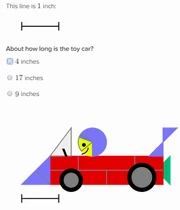FANDOM

1,895 Pages

Estimate lengths
Description
Exercise Name: Estimate lengths
Math Missions: Early math Math Mission
Types of Problems: 1

The Estimate lengths exercise appears under the Early math Math Mission. This exercise practices mentally manipulating lengths and using them to find the lengths of other related objects and sides.

Types of Problems

There is one type of problem in this exercise:

1. Use the length to estimate another: This problem provides a length, or a key, and an object to be measured. The student is expected to take the given unit and use it to estimate the length of some part of the given object. The answer is to be selected from a multiple choice list.Use the length to estimate another

Strategies

Knowledge of numbers and ratios and ability to move lengths are encouraged to ensure success on this exercise.

1. A make-shift ruler, like a piece of paper or a pencil, can be used to estimate the lengths by comparison.
2. The answer will be obvious if done correctly, so if there are two "close" answers among the choices these are often not the answer.

Real-life Applications

1. Estimating lengths mentally is a resource for checking answers in higher maths.
2. This skill is useful for understanding numbers as quantity.
Community content is available under CC-BY-SA unless otherwise noted.